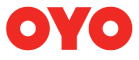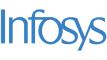New update is available. Click here to update.

# Next Permutation

Medium0/80
15 mins
85 %335 upvotes+17 more

## Problem Statement

#### For example, [2, 1, 3, 4] is lexicographically smaller than [2, 1, 4, 3].

Detailed explanation ( Input/output format, Notes, Images )
##### Constraints:
``````1 <= T <= 50
1 <= N <= 10000
1 <= P[i] <= N

Time limit: 1 sec
``````
##### Sample Input 1:
``````2
3
1 2 3
5
2 3 1 4 5
``````
##### Sample Output 1:
``````1 3 2
2 3 1 5 4
``````
##### Explanation of sample input 1:
``````In the first test case, the lexicographically next greater permutation is [1, 3, 2].

In the second test case, the lexicographically next greater permutation is [2, 3, 1, 4, 5].
``````
##### Sample Input 2:
``````2
2
1 2
3
3 1 2
``````
##### Sample Output 2:
``````2 1
3 2 1
``````
##### Explanation for sample input 2:
``````In the first test case, the lexicographically next greater permutation is [2, 1].

In the second test case, the lexicographically next greater permutation is [3, 2, 1].
``````AutocompleteConsole